## CodeNewbie Community 🌱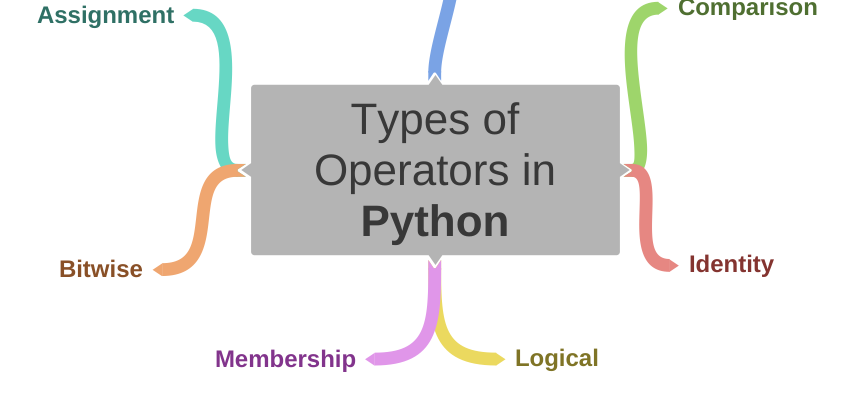Aswin Barath

Posted on • Originally published at hackernoon.com

# 7 Types of Operators in Python

Operators are used to doing operations on any given data stored inside variables. In Python, we learn 7 types of operators - namely :

1. Arithmetic operators
2. Bitwise operators
3. Comparison operators
4. Assignment operators
5. Logical operators
6. Identity operators
7. Membership operators

## 1. Arithmetic Operators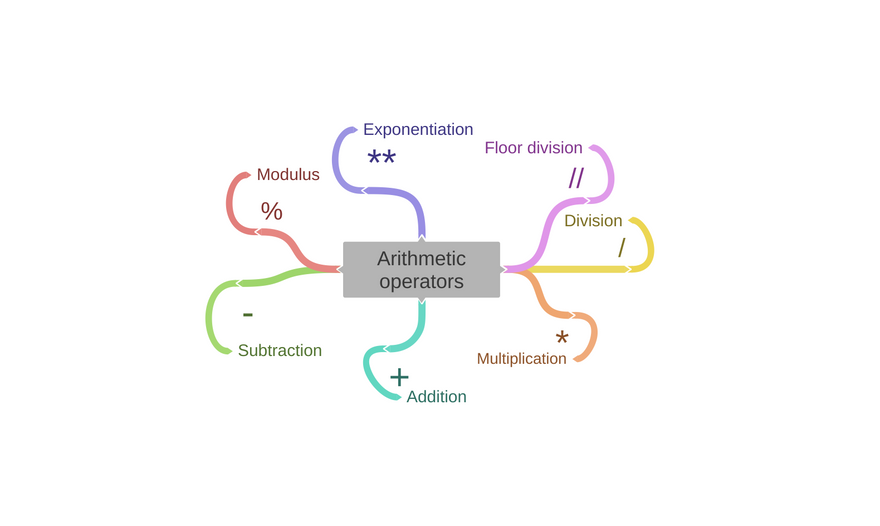Arithmetic operators makes mathematical operations possible on operands in a program.

I know! I know! This is a basic concept! But let's make it fun!

## Starting with addition and subtraction

``````Addition 9
Subtraction 8
``````

## Now, Multiplication and Division

``````Multiplication 314.0
Division 2.0
``````

## Special arithmetic operators

• Floor division - `//`: rounds of the result to the nearest whole number.
• Modulus - `%`: produces the remainder of the numbers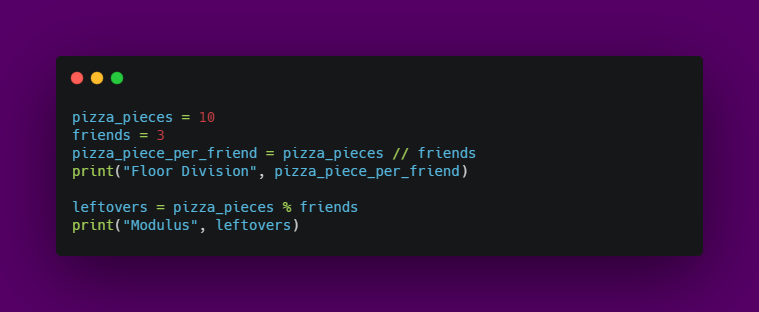Output:
``````Floor Division 3
Modulus 1
``````
• Exponentiation - ** : produces the power of given numbers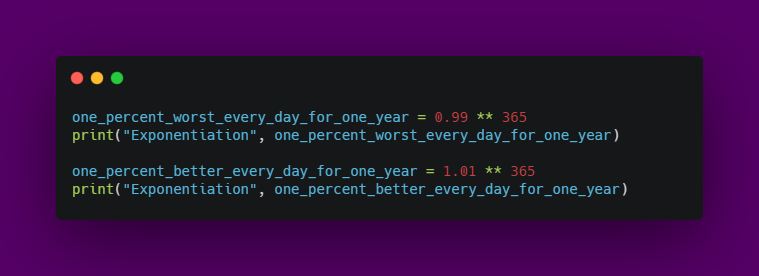Output:
``````Exponentiation 0.025517964452291125
Exponentiation 37.78343433288728
``````

## 2. Bitwise operators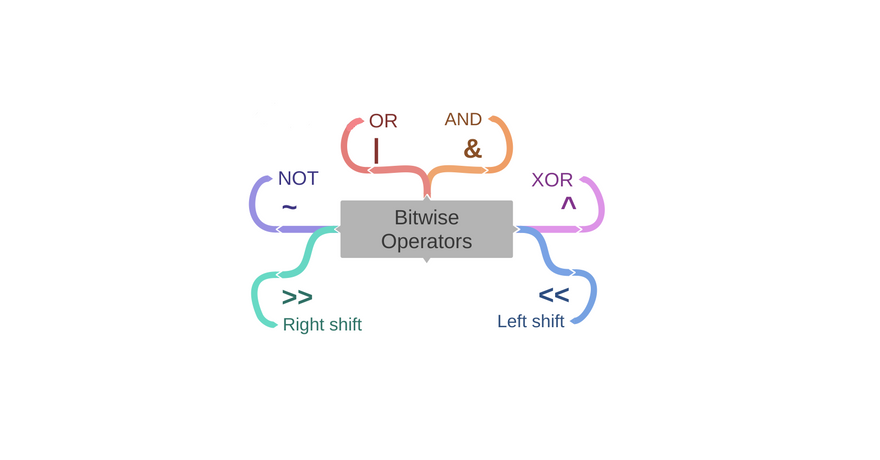When it comes to binary numbers, bitwise operators are the choice.

Bitwise operators are used to performing operations on binary numbers.

## AND, OR, XOR operators

• AND `&` operator sets each bit to 1 if both bits are 1.
• OR `|` operator sets each bit to 1 if one of two bits is 1.
• XOR `^` operator sets each bit to 1 if only one of two bits is 1.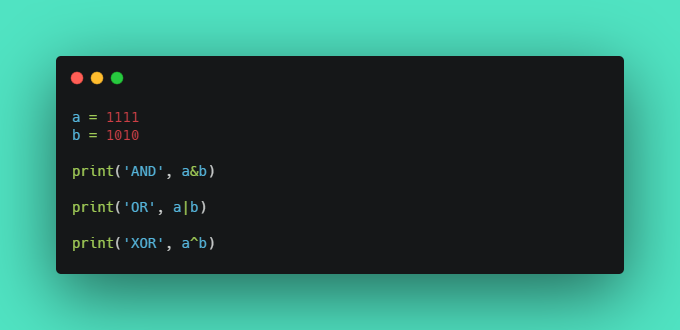Output:
``````AND 82
OR 2039
XOR 1957
``````

Ha Ha, surprised about the outputs?!
The outputs are a result of the binary numbers a and b which gets converted into an integer, each time bitwise operation is performed.

## NOT operator

• NOT `~` operator inverts all the bits.
• In python, the number gets converted into an inverted signed number.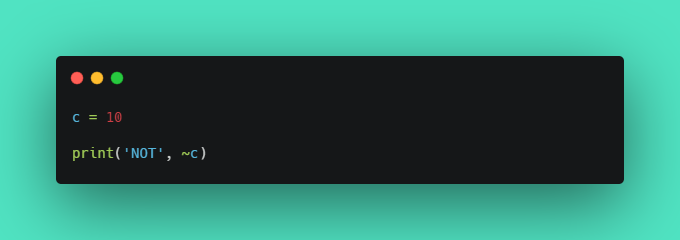Output:
``````NOT -11
``````

## Shift operators

• left shift `<<` operator shifts left by pushing zeros in from the right and let the leftmost bits fall off.
• right shift `>>` operator shifts right by pushing copies of the leftmost bit in from the left, and let the rightmost bits fall off.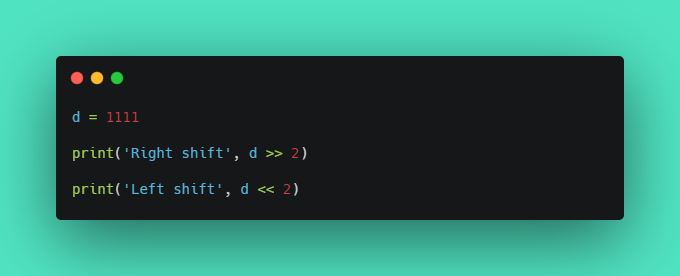Output:
``````Right shift 277
Left shift 4444
``````

## 3. Comparison Operators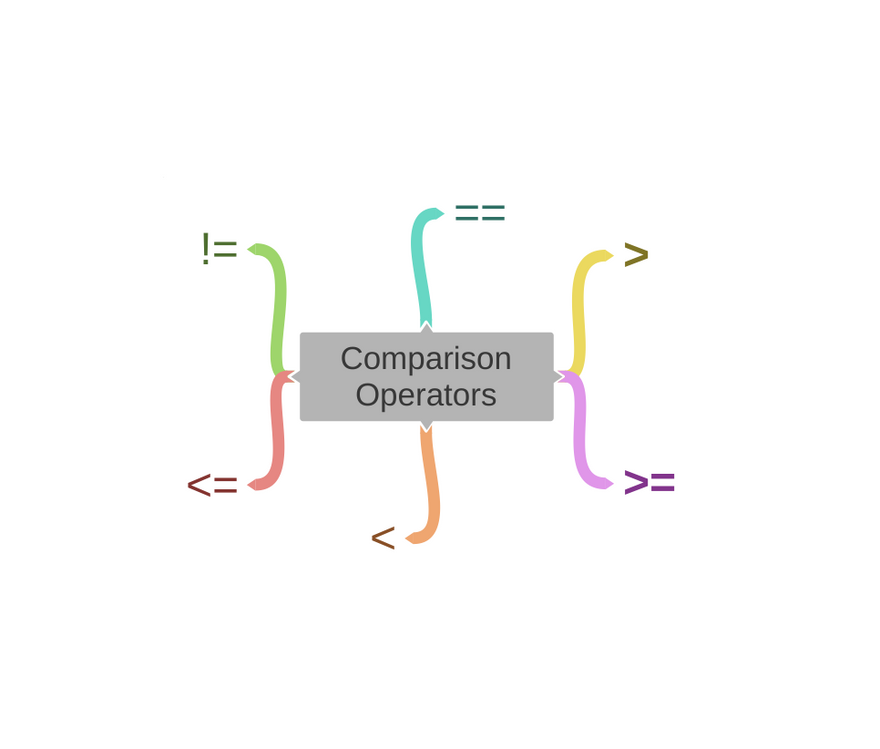So, basically, comparison operators are used to comparing two values that are numbers.

If we level up to be geeky, comparison operators can also be used to compare other data types.

Now, let's start with equality checks and I hope you like spider-man movies.

## `==` Equal comparison operator

``````False
True
``````

## `!=` Not Equal comparison operator

``````True
False
``````

Alright, I'm sure that you are aware of how to use other operators to compare two number values, right?
OK, now's the time to level up to be geeky.

For the rest of the operators let us compare the letters from the Alphabet.
Wait, what?!
You heard me right!

Let me explain it at the end of this post.

## `>` Greater than comparison operator

``````False
True
False
``````

## `<` Less than comparison operator

``````True
True
False
``````

## `>=` Greater than or equal to comparison operator

``````False
True
True
``````

## `<=` Less than or equal to comparison operator

``````False
True
True
``````

Here's the answer for the above craziness.

When we compare two letters (or characters), it gets converted into ASCII code. You can check the link where the table contains 'DEC' (Decimal values) for the characters from Alphabet.

Now that the characters are converted into ASCII code, which is nothing but numbers and we are back to square one.
That is, we can compare the values as numbers and return True or false.

## 4. Assignment Operators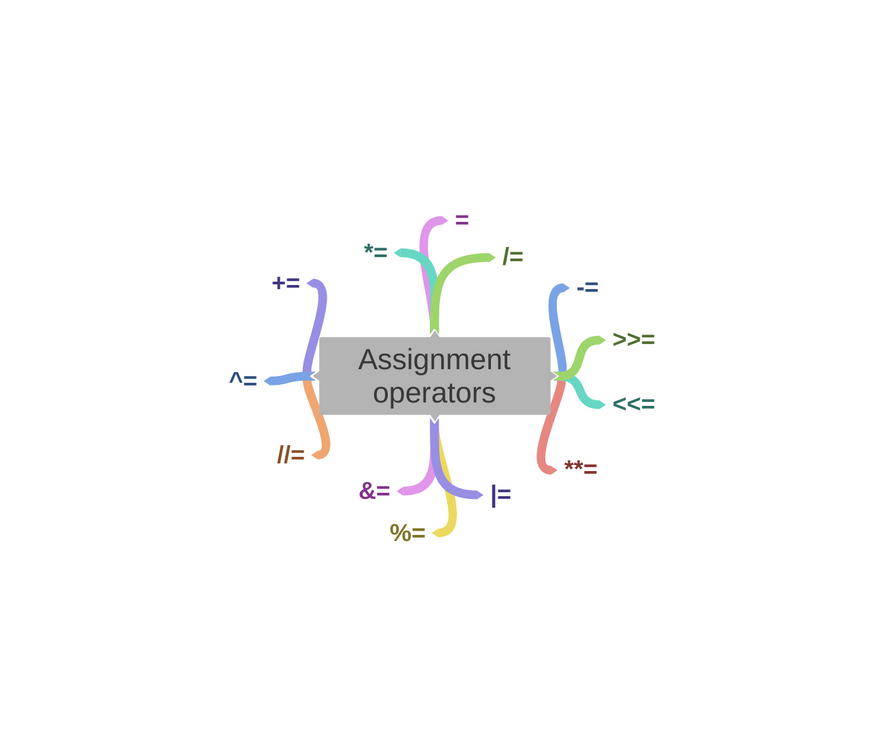Assignment operators are used to assigning values to variables.

That is to store values in variables we use `=` assignment operator.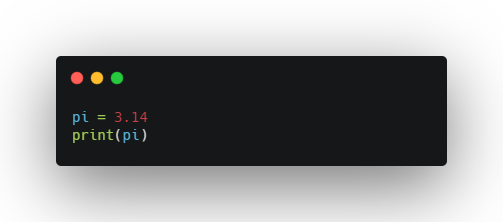Output:

``````3.14
``````

OK, now comes the real fun.
Have ever been tired to use `x = x + 5`, where we type the variable x twice?
There's actually a shortcut for this called Augmented assignment operators.

Augmented assignment operators can be used as a replacement as follows:

``````x += 3     --->    x = x + 3
x -= 3     --->    x = x - 3
x *= 3     --->    x = x * 3
x /= 3     --->    x = x / 3
x %= 3     --->    x = x % 3
x //= 3    --->    x = x // 3
x **= 3    --->    x = x ** 3
x &= 3     --->    x = x & 3
x |= 3     --->    x = x | 3
x ^= 3     --->    x = x ^ 3
x >>= 3    --->    x = x >> 3
x <<= 3    --->    x = x << 3
``````

## Here's the Code and Output

``````9
6
18
6.0
``````
``````64
1
0
``````
``````2
3
``````
``````0
3
24
``````

Quick Note: The code snippets reuses the same variable to assign with different arithmetic operations / bitwise operations / shift operations.

So, while coding makes sure you practice to use print statements after each operation.

## 5. Logical operators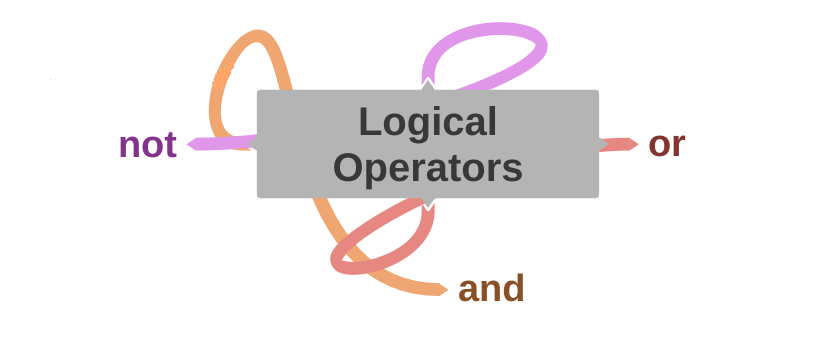Logical operators are used to combining more than two conditional statements.

These operators are very useful for writing logical conditions in control flow statements of a programming language.

Let's code them one by one.

## `and` operator

• and operator return the boolean value `True` only if both statements are true.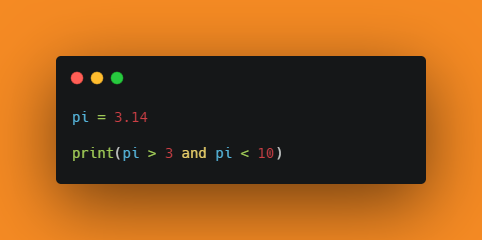Output:
``````True
``````

## `or` operator

• or operator returns the boolean value `True` if any statement is true.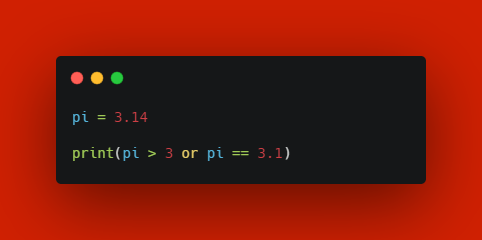Output:
``````True
``````

## `not` operator

• not-operator acts as a unary operator which returns the boolean value `True` the statement is true and vice versa.Output:
``````False
``````

## 6. Identity Operators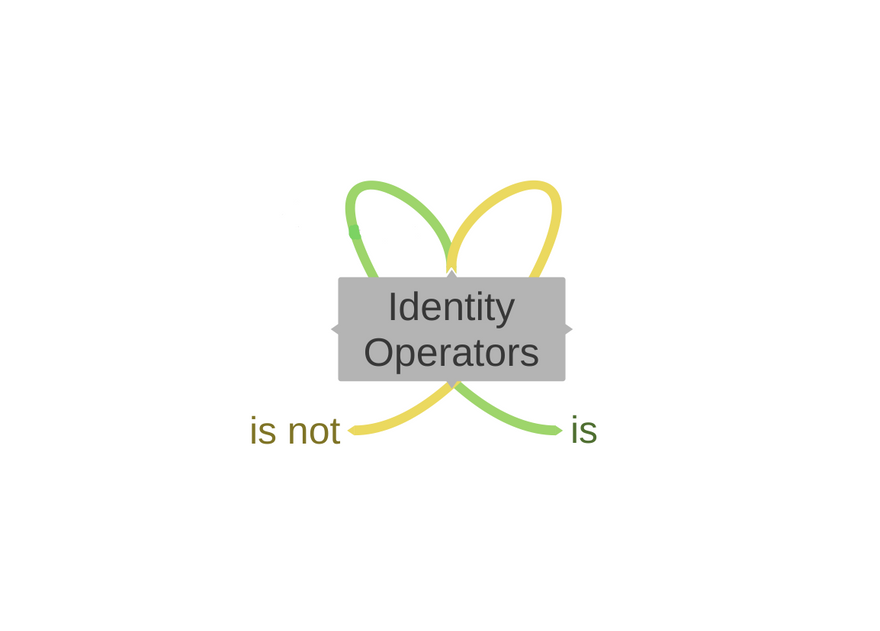Identity operators are used to checking whether the objects are the same or not.

Fact: In python, all data types are implemented as an object.

## `is` operator

``````True
False
True
``````

## `is not` operator

``````True
False
True
``````

## 7. Membership Operators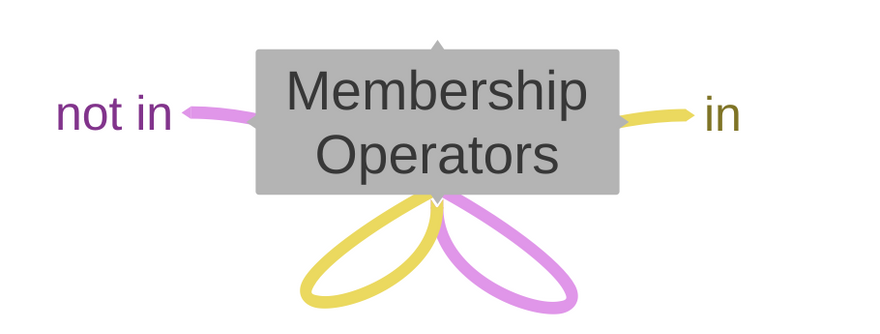Membership operators are used to testing if a sequence with the specified value is present in the given object.

Fact: In python, all data types are implemented as an object.

Let's go code through each of them.

## `in` operator

``````True
``````

## `not in` operator

``````True
``````